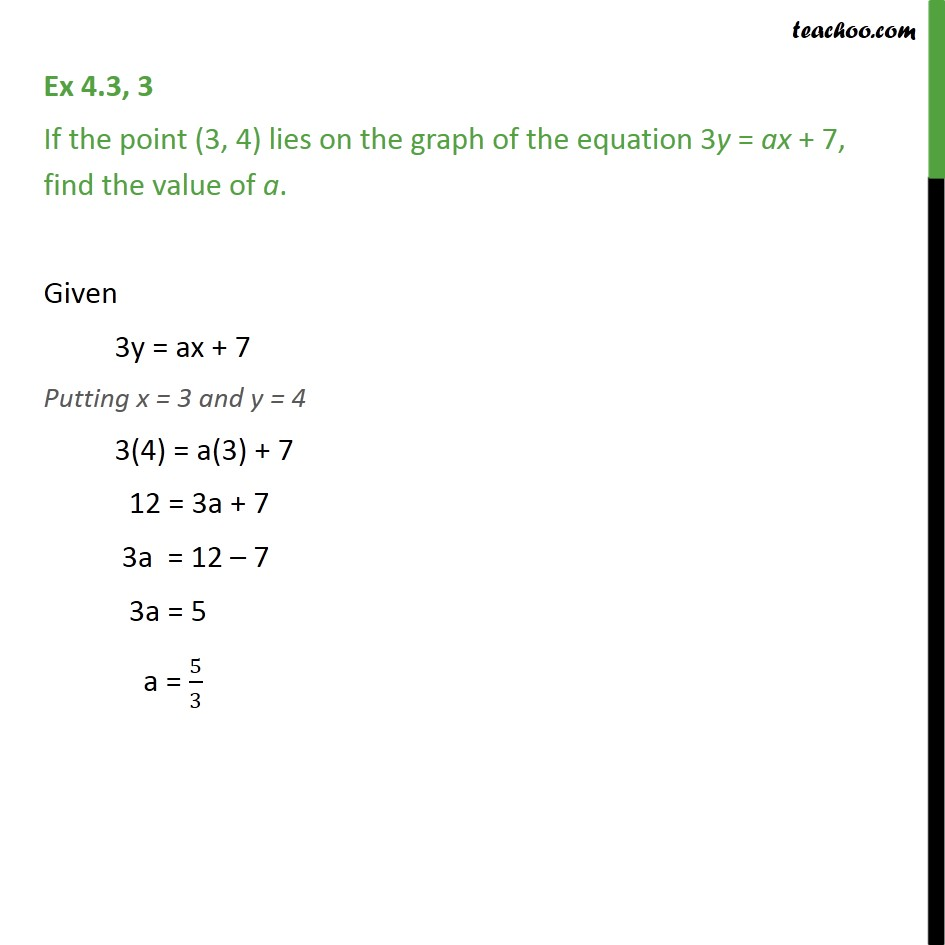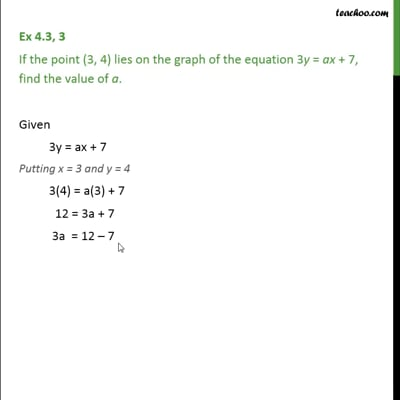Ex 4.3

Chapter 4 Class 9 Linear Equations in Two Variables
Serial order wiseThis video is only available for Teachoo black users

Maths Crash Course - Live lectures + all videos + Real time Doubt solving!

### Transcript

Ex 4.3, 3 If the point (3, 4) lies on the graph of the equation 3y = ax + 7, find the value of a. Given 3y = ax + 7 Putting x = 3 and y = 4 3(4) = a(3) + 7 12 = 3a + 7 3a = 12 – 7 3a = 5 a = 5/3